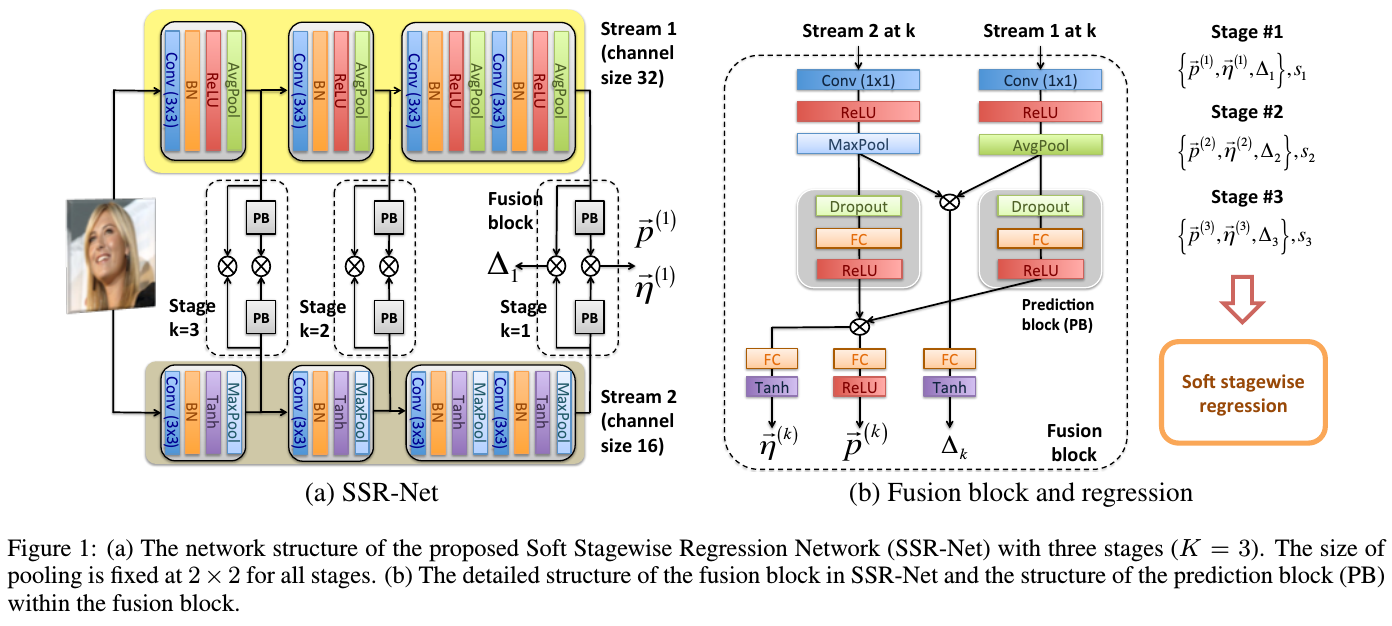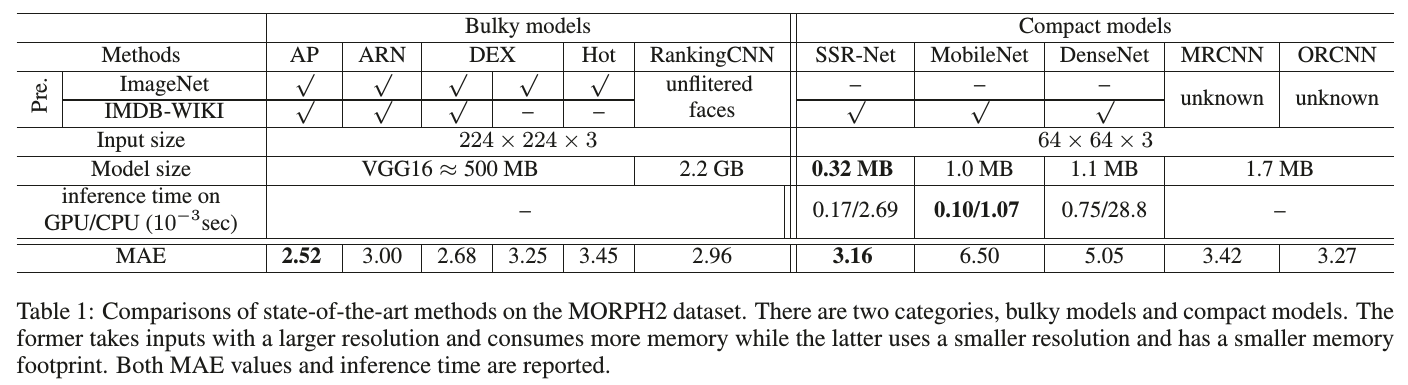# 论文阅读-年龄估计_SSRNet

### 2. 庐山真面DEX 将年龄均分为 $s$ 个年龄段，即对于[0,V]的年龄跨度，每个年龄段跨度为 $V/s$，该段的代表性年龄取 $\mu = V/s$.

$\tilde{y} = \vec{p}\cdot \vec{\mu}=\sum_{i=0}^{s-1}p_i\cdot\mu_i=\sum_{i=0}^{s-1}p_i\cdot i(\frac{V}{s})$

multi-stage prediction.”

$\tilde{y} =\sum_{k=1}^{K} \vec{p}^{(k)}\cdot \vec{\mu}^{(k)}=\sum_{k=1}^{K}\sum_{i=0}^{s_k-1}p_i^{(k)}\cdot i(\frac{V}{\prod_{j=1}^k s_j })$

$\bar{s}_k=s_k \cdot (1+\bigtriangleup_k)$

$\bar{w}_k=\frac{V}{\prod_{j=1}^k \bar{s}_j}$

$\bar{i}=i +\eta^k_i$

$\tilde{y}=\sum_{k=1}^{K}\sum_{i=0}^{s_k-1}p_{i}^{k}\cdot \bar{i}(\frac{V}{\prod_{j=1}^k\bar{s}_j})$### 3. 顾盼生姿regards.

#### oukohou.©️2019 CSDN 皮肤主题: 大白 设计师: CSDN官方博客